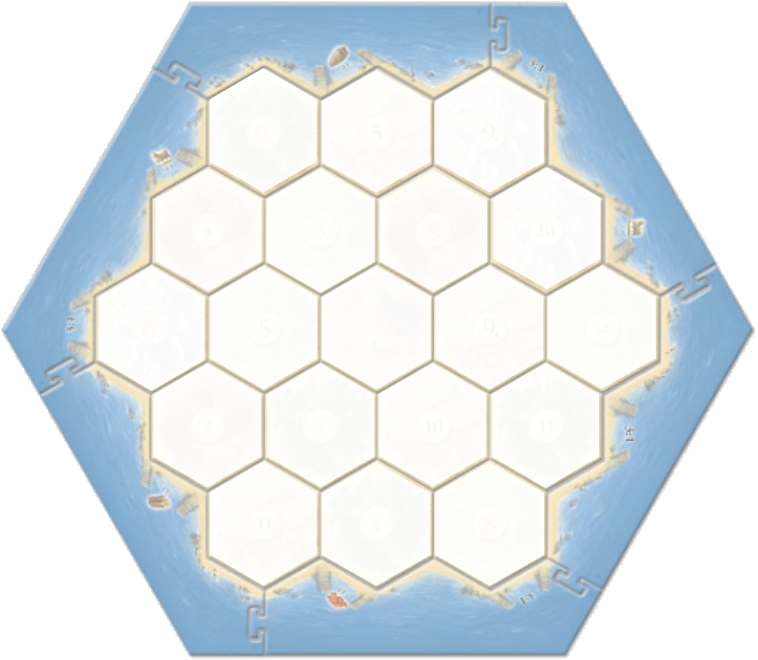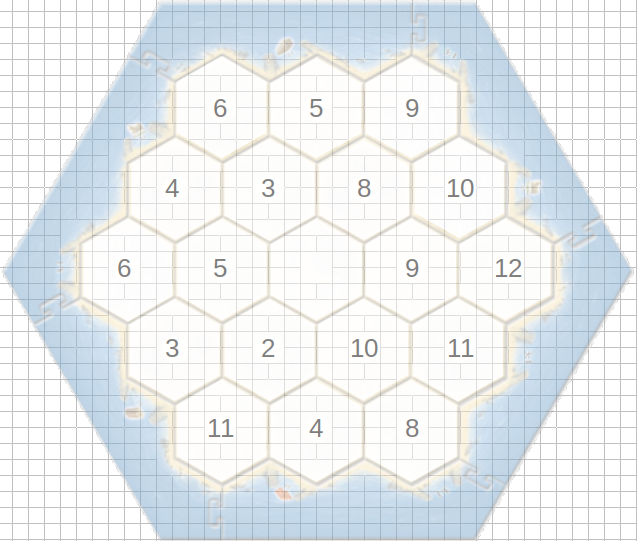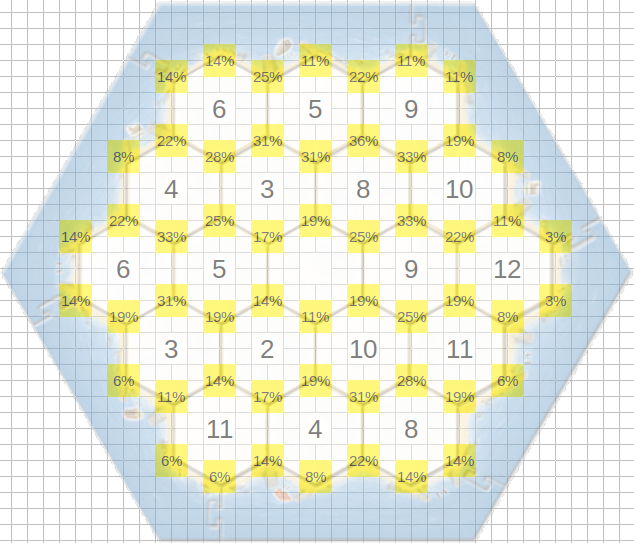# What is the chance of earning resources in this round?

Catan is a very popular board game, known mainly for the configuration of its board to be assembled from hexagons and also for its dynamics involving the negotiation of resources among other players for constructions and developments within the game. The classic version board consists of 19 hexagons arranged as in the figure below.Arranged above each hexagon is an element of the following numerical set (without repetition):

{2; 3; 3; 4; 4; 5; 5; 6; 6; 8; 8; 9; 9; 10; 10; 11; 11; 12}.To understand the dynamics of the game a little, we have 5 types of raw materials that are necessary to carry out the constructions and developments. Each hexagon produces a single type of raw material, and all players with villages or cities located at one of the vertices of the referent hexagon, will receive one (if they have a village) or two (if they have a city) resources from that region, when the sum of the two conventional 6-sided dice cast corresponds to the number in the center of the hexagon.

With this it is already possible to realize that a region will be left with no number (we have 19 regions and 18 numbers). In the classic game this region is represented by the desert and it does not generate any resources.

Analyzing the occurrences of each data pair, we can determine among the other numbers, how many times they occur.

1 sum occurrence = 2 → {1,1}

2 sum occurrences = 3 → {2,1}; {1,2}

3 sum occurrences = 4 → {3,1}; {2,2}; {1,3}

4 sum occurrences = 5 → {4,1}; {3,2}; {2,3}; {1,4}

5 sum occurrences = 6 → {5,1}; {4,2}; {3,3}; {2,4}; {1,5}

5 sum occurrences = 8 → {6,2}; {5,3}; {4,4}; {3,5}; {4,6}

4 sum occurrences = 9 → {6,3}; {5,4}; {4,5}; {3,6}

3 sum occurrences = 10 → {6,4}; {5,5}; {4,6}

2 sum occurrences = 11 → {6,5}; {5,6}

1 sum occurrences = 12 → {6,6}

In all, we have 30 occurrences referring to the numbers spread across the regions of the map, and 6 occurrences whose sum would give 7, which despite having a meaning in the game, will not be relevant for this discussion. In the game, no village can be built on a vertex that is less than two edges from another village. Thus, for each village built, it blocks the construction of other villages at up to 4 vertices (one vertex on which the village itself was built and up to three other vertices connected to an edge of that vertex).

The game starts with the first player building a village in any position on the board, then the second player, thus up to the last player. Then the last player builds a new village, then the penultimate player builds a new village, so even the first player.

Considering a match with 3 players, we will have:

1st player – 1st time: you have exactly 54 options;

2nd player – 1st time: have at least 50 options;

3rd player – 1st time: has at least 46 options;

3rd player – 2nd time: has at least 42 options;

2nd player – 2nd time: has at least 38 options;

1st player – 2nd time: has at least 32 options;

Considering a match with 4 players, we will have:

1st player – 1st time: you have exactly 54 options;

2nd player – 1st time: have at least 50 options;

3rd player – 1st time: has at least 46 options;

4th player – 1st time: has at least 42 options;

4th player – 2nd time: has at least 38 options;

3rd player – 2nd time: has at least 34 options;

2nd player – 2nd time: have at least 30 options;

1st player – 2nd time: has at least 26 options;

In both situations, we have many options to choose from, but someone who has played Catan could answer that despite many options, the number of “interesting” options is not so great. However, when inserting the adjective “interesting” to an option, we are assigning some characteristics that can be measured, to define by a criterion which option is “most interesting”.

In a recent game, I was asked how to calculate the probability that in a play we will obtain resource at a vertex. The solution is quite simple, if a vertex had connected to 11 regions with numbers ranging from 2 to 12 (including 7), every vertex this vertex would receive resources. That is, your probability would be 100%.

Thus, the probability of each vertex yielding a resource in that round is given by the sum of the individual probabilities of each region around it to generate resources in that round, less the repetition of the regions.

In this case, imagine a vertex connected to a region with the number 5. This number occurs in 4 different ways, among the 36 possible ones. Thus, 4/36 represents the probability of obtaining a 5, something close to 11%.

If our vertex is connected to another number 5. The probability of obtaining resources in this round will not be the sum of the two regions generating resources. Because the new region does not expand the space of occurrences. So, connected to two regions with the number 5, we continue with 4/36 of the occurrences, what changes in this case is the weight of the resources obtained in those occurrences, which will be 2 for a village and 4 for a city (considering any resource as weight 1).More generally, we can define the maximum probability of a vertex, being between regions of numbers 6, 8 and 9 or 5, 6 and 8. In both cases we obtain 14/36 of the cases, equivalent to 39%.

The figure on the previous page shows the probabilities of each vertex for the arrangement already presented. It was generated in a common spreadsheet using the reasoning discussed in this chapter (the most difficult thing was to hit the background image with the spreadsheet cells).

But choosing a vertex is something more complex to understand than just the probability that each position will yield resources. This is a much more difficult problem (this ignoring resources and ports), because as we discussed earlier, choosing a vertex implies blocking up to three other vertices. In this way a choice can be made not for its own benefit, but for mutual harm. We can, for example, opt for a position whose probability of generating resources in that round is not so great, but which at the same time blocks three other vertices with a great chance of generating resources. Thus, this is a choice that must take into account several other factors, which would avoid the discussion of this humble text.